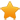Switch Editions?
CancelChannel: ClearScript
Viewing all articles

# New Post: Passing javascript objects to c# native code

0
0
Thanks for the warning. I wanted to know just how expensive the dynamic statement was - so I ran a test. The Console.Write was 3-4 times as fast, but to be fair, I added a third way "console.logs" deserializes a JavaScript literal and uses the same recursive printing style as console.log.
``````public class Konsoll
{
public int Indent = 0;
public JavaScriptSerializer jss = new JavaScriptSerializer();
public void logs(string s)
{
object o = jss.DeserializeObject(s);
loggs(0, o);
}
private void loggs(int indent, object o)
{
if (o is Dictionary<string, object>)
{
foreach (KeyValuePair<string, object> kvp in (Dictionary<string, object>)o )
{
Console.Write(kvp.Key);
Console.Write(':');
loggs(indent + Indent, kvp.Value);
}
}
else if (o is Object[])
{
for (int i = 0; i < ((Object[])o).Length; i++)
{
Console.Write(i);
Console.Write(':');
loggs(indent + Indent, ((Object[])o)[i]);
}
}
else if (o == null)
{
Console.Write("null");
}
else
{
Console.Write(o.ToString());
}

}
private void logg(int indent, object o)
{

if (o is System.Dynamic.DynamicObject)
{

foreach (string egenskap in ((System.Dynamic.DynamicObject)o).GetDynamicMemberNames())
{
// Console.Write(new String(' ', indent));
Console.Write(egenskap);
Console.Write(':');
logg(indent + Indent, ((dynamic)o)[egenskap]);
}
}
else if (o == null)
{
Console.Write("null");
}
else
{
Console.Write(o.ToString());
}
}
public void log(object o)
{
logg(0, o);
}

}``````
Writing a test:
``````            using (var engine = new V8ScriptEngine())
{
var start3 = DateTime.Now;
for (int i = 0; i < 1000; i++)
{
engine.Execute("Console.Write(JSON.stringify({v1:4,v2:['a','bc', 'de', {f:'g'}],h:1,i:2,j:3,k:4}));");
}
var tid3 = DateTime.Now.Subtract(start3);

var start = DateTime.Now;
for (int i = 0; i < 1000; i++ )
{
engine.Execute("console.logs(JSON.stringify({v1:4,v2:['a','bc', 'de', {f:'g'}],h:1,i:2,j:3,k:4}));");
}
var tid = DateTime.Now.Subtract(start);
var start2 = DateTime.Now;
for (int i = 0; i < 1000; i++)
{
engine.Execute("console.log({v1:4,v2:['a','bc', 'de', {f:'g'}],h:1,i:2,j:3,k:4});");
}
var tid2 = DateTime.Now.Subtract(start2);

Console.WriteLine("static tok : " + tid.ToString());
Console.WriteLine("dynamic tok : " + tid2.ToString());
Console.WriteLine("Console.Write tok: " + tid3.ToString());
string str;
while ((str = Console.ReadLine()) != "end")
{
engine.Execute(str);
}
}
``````
Getting the result:
``````static tok : 00:00:05.3335333
dynamic tok : 00:00:05.6545654
Console.Write tok: 00:00:02.4172417``````
So the "static" console.logs alternative to dynamic only slightly cheaper.

In the logging example - I should just pass the jsonstring to .net, but generally, if I need to send a literal to .net in a predefined structure, it's ok to use dynamic. I think, however, there is one weakness in GetDynamicMemberNames: that you don't know if its an array or object. Is there another way of knowing?

Viewing all articles

More Pages to Explore .....